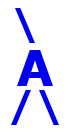## AIRES library reference: intdati.

```c
c     Routine directly callable from C/C++ (it could be necessary to
c     append an underscore, "_", to its name depending on the C/C++
c     compiler used).
c
c
subroutine intdati(idati)
c
c     Current date and time as integer numbers.
c     SYSTEM DEPENDENT CALL INSIDE!
c
c     Written by: S. J. Sciutto, La Plata 1996.
c
c     Parameters:
c     ==========
c
c     idati........... (output, integer, array(6)) Array containing
c                      the following data:
c                         idati(1) = year (yyyy).
c                         idati(2) = month (1 to 12)
c                         idati(3) = day (1 to 31)
c                         idati(4) = hour (1 to 24)
c                         idati(5) = minutes (0 to 59)
c                         idati(6) = seconds (0 to 59)
c
c
c<--->
```

 These pages are maintained by Sergio J. Sciutto Back to AIRES library index Back to AIRES home